# LOAD & RESISTANCE FACTOR DESIGN (LRFD)Reading time: 1 minute

#### Load and Resistance Factor Design FormatOf the many multiple safety factor formats in vogue, perhaps the simplest to understand is the Load and Resistance Factor Design (LRFD) format, which is adopted by the ACI Code [Ref. 3.5, 3.8, 3.9]. Applying the LRFD concept to the classical reliability model, adequate safety requires the following condition to be satisfied :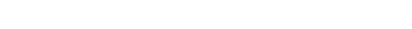where Rn and Sn denote the nominal or characteristic values of resistance R and load effect S respectively;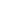and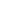denote the resistance factor and load factor respectively. The resistance factoraccounts for ‘under-strength’, i.e., possible shortfall in the computed ‘nominal’ resistance, owing to uncertainties related to material strengths, dimensions, theoretical assumptions, etc., and accordingly, it is less than unity. On the contrary, the load factor, which accounts for ‘overloading’ and the uncertainties associated with Sn, is generally greater than unity.

Eq. 1 may be rearranged as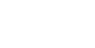which is representative of the safety concept underlying WSM,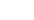here denoting the ‘factor of safety’ applied to the material strength, in order to arrive at the permissible stress for design. Alternatively, Eq. 1 may be rearranged as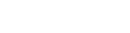which is representative of the safety concept underlying ULM,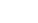here denoting the so-called ‘load factor’ (ULM terminology) applied to the load in order to arrive at the ultimate load for design.